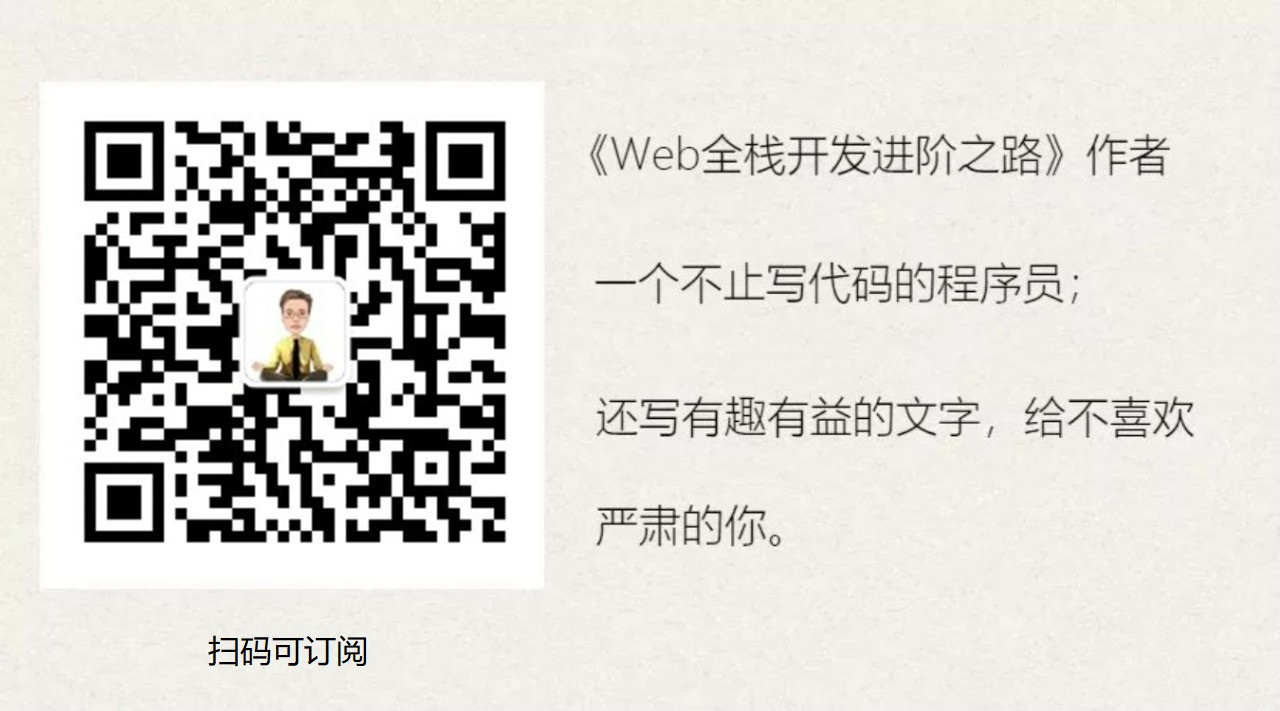# 【面试】如果你这样回答“什么是线程安全”，面试官都会对你刮目相看

2019.05.09 - 转载自李新杰的博客园

### 私有的东西就不该让别人知道

``````double avgScore(double[] scores) {
double sum = 0;
for (double score : scores) {
sum += score;
}
int count = scores.length;
double avg = sum / count;
return avg;
}
``````

### 大家不要抢，人人有份

``````class StudentAssistant {

String determineDegree() {
double score = totalScore.get();
if (score >= 90) {
return "A";
}
if (score >= 80) {
return "B";
}
if (score >= 70) {
return "C";
}
if (score >= 60) {
return "D";
}
return "E";
}

double determineOptionalcourseScore() {
double score = totalScore.get();
if (score >= 90) {
return 10;
}
if (score >= 80) {
return 20;
}
if (score >= 70) {
return 30;
}
if (score >= 60) {
return 40;
}
return 60;
}
}
``````

### 只能看，不能摸

``````class StudentAssistant {

final double passScore = 60;
}
``````

### 没有规则，那就先入为主

``````class ClassAssistant {

double totalScore = 60;
final Lock lock = new Lock();

void addScore(double score) {
lock.obtain();
totalScore += score;
lock.release();
}

void subScore(double score) {
lock.obtain();
totalScore -= score;
lock.release();
}
}
``````

### 相信世界充满爱，即使被伤害- END -

430